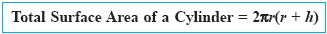Name: ___________________Date:___________________

kwizNET Subscribers, please login to turn off the Ads!
 Email us to get an instant 20% discount on highly effective K-12 Math & English kwizNET Programs!

### High School Mathematics4.60 Total Surface Area of a Cylinder

 Total Surface Area of a cylinder = Lateral surface area + Area of two circles (top and bottom circles)Total surface area = 2*pi*r2 + 2*pi*r*h = 2 * pi * r * (r+h).Example: The ratio of the radius and height of a cylinder is 5:3. The radius is 25cm. Find its total surface area. Solution: Given that r = 25cm. Height (h) = 3/5 * 25 = 15cm. Total surface area = 2 * pi * r * (h+r). = 2 * 22/7 * 25(25+15) = 1100/7 * 40 = 6285.71 Sq. cm. Directions: Solve the following problems and write the answer in two decimal places. Also write at least 5 examples of your own.
 Q 1: The ratio of the radius and height of a cylinder is 7:5. The radius is 35cm. Find its total surface area.13200 Sq. cm.13600 Sq. cm.13400 Sq. cm.13800 Sq. cm. Q 2: The ratio of the radius and height of a cylinder is 5:6. The radius is 25cm. Find its total surface area.8648.86 Sq. cm.8642.86 Sq. cm.8644.86 Sq. cm.8646.86 Sq. cm. Q 3: The ratio of the radius and height of a cylinder is 4:3. The radius is 16cm. Find its total surface area.2182 Sq. cm.2184 Sq. cm.2186 Sq. cm.2188 Sq. cm. Q 4: The ratio of the radius and height of a cylinder is 4:3. The radius is 24cm. Find its total surface area.6332 Sq. cm.6334 Sq. cm.6338 Sq. cm.6336 Sq. cm. Q 5: The ratio of the radius and height of a cylinder is 3:4. The radius is 21cm. Find its total surface area.6468 Sq. cm.6464 Sq. cm.6462 Sq. cm.6466 Sq. cm. Q 6: The ratio of the radius and height of a cylinder is 7:5. The radius is 21cm. Find its total surface area.4758 Sq. cm.4752 Sq. cm.4756 Sq. cm.4754 Sq. cm. Question 7: This question is available to subscribers only! Question 8: This question is available to subscribers only!

#### Subscription to kwizNET Learning System costs less than \$1 per month & offers the following benefits:

• Unrestricted access to grade appropriate lessons, quizzes, & printable worksheets
• Instant scoring of online quizzes
• Progress tracking and award certificates to keep your student motivated
• Unlimited practice with auto-generated 'WIZ MATH' quizzes
• Child-friendly website with no advertisements

© 2003-2007 kwizNET Learning System LLC. All rights reserved. This material may not be reproduced, displayed, modified or distributed without the express prior written permission of the copyright holder. For permission, contact info@kwizNET.com
For unlimited printable worksheets & more, go to http://www.kwizNET.com.